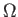Next: Preliminaries Up: Lower Query Bounds in Previous: Nonconstant Symmetric Functions   Contents

# Graph Properties

In the previous chapter we proved lower query bounds for Boolean functions whose outputs show symmetry with respect to the Hamming weight of the inputs. We now consider lower bounds for graph properties. In Section 3.1 we cover the definitions of deterministic decision trees, evasiveness and graph properties, and formalize how we will represent graphs as bit strings so that we may examine them in the oracle query model. We also present the Aanderaa-Karp-Rosenberg conjecture, which motivates the study of non-trivial monotone graph properties in particular.

We apply the result of Section 2.5 with limited success to generic non-trivial monotone graph properties in Section 3.2. We then apply Ambainis' Theorem 2.1.1 to attain new lower bounds of(V) for computing connectivity and bipartiteness in Sections 3.2.1 and 3.2.2. The lower bounds attained for these problems leads us to question if there is a quantum extension of the Aanderaa-Karp-Rosenberg conjecture in the quantum bounded error setting, in Section 3.3 we establish that there is not.

SubsectionsNext: Preliminaries Up: Lower Query Bounds in Previous: Nonconstant Symmetric Functions   Contents
Matthew Hayward Lower Query Bounds in the Quantum Oracle Model GitHub Repository